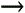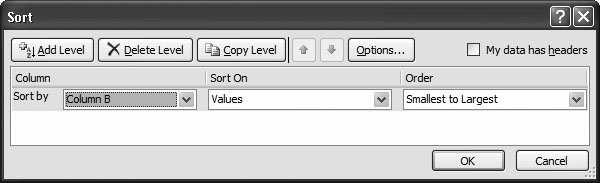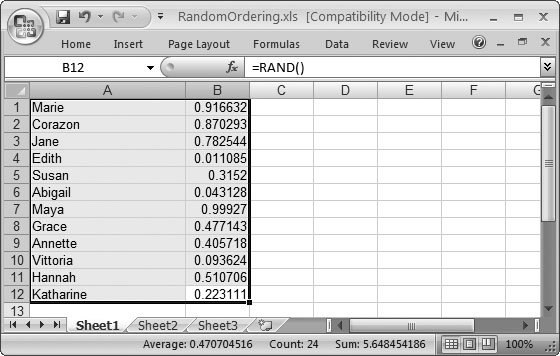Section 9.3. General Math Functions

9.3. General Math Functions

Excel's Math & Trig category contains functions that go a step beyond the basic mathematical operators (like +,, /, *, and ^) to help you figure out things like absolute values and greatest (and least) common denominators.

Tip: Poker fan alert: the COMBIN( ) and PERMUT( ) functions may help you keep your game sharp even when you're away from the table.

This section covers 14 of the most commonly used functions in this category.

9.3.1. PRODUCT( ), FACT( ), POWER( ), and SQRT( ): Products, Factorials, Powers, and Square Roots

These four functions actually offer the same services provided by many of the simple operators covered in the last chapter, but, in some cases, they come with a few twists that may save you time.

The PRODUCT( ) function takes a list of numbers , multiplies them together, and gives the result. For example, the following formula multiplies 2*3*3, and arrives at 18:

=PRODUCT(2,3,3)

One interesting characteristic of the PRODUCT( ) function is that in addition to accepting individual cell references, it also supports cell ranges . You can use PRODUCT( ) to multiply all the numbers in a range in the same way you use SUM( ) to add numbers. Here's an example:

=PRODUCT(A2:A12)

You can use the FACT( ) function to calculate a factorial , which is a sequence of numbers (starting at 1), each of which is multiplied together to arrive at the result. For example, 5! (pronounced "five factorial") translates into 5*4*3*2*1, which works out to 120. Rather than typing in all these numbers and their accompanying multiplication symbols, you can use the following convenient formula:

=FACT(5)

People use factorials in many areas of math, including probability theory and calculus. On the other hand, you aren't likely to find factorials cropping up in financial statements or expense reports .

POWER( ) is a straightforward function used for working with exponents. For example, if you want to calculate 4 3 , you can use one of two approaches. You can use the exponentiation operator:

=4^3

or you can opt for the POWER( ) function:

=POWER(4,3)

The POWER( ) function is useful if you frequently use cube rootsor any other exponential expressions whose exponent is a fractionbecause it lets you write clearer formulas. For example, imagine you want to find the cube root of 4, which, on paper, would be written as 4 1/3 . Here's the operator-based expression for that:

=4^(1/3)

With the POWER( ) function, you don't need to use the parentheses (and, more important, you have no chance of getting the wrong answer because you forgot to use them):

=POWER(4,1/3)

If you simply want to calculate the square root of a number, you can use the POWER( ) function, or you can summon the SQRT( ) function, which requires only a single argument. Here's a sample formula that gives you 3:

=SQRT(9)

9.3.2. QUOTIENT( ) and MOD( ): Higher Division

QUOTIENT( ) and MOD( ) (see Figure 9-7) are handy for fancy division problems.Figure 9-7. The QUOTIENT( ) and MOD( ) functions let you break a number down into two pieces. In this case, the worksheet uses QUOTIENT( ) to find out how many pizzas you can afford with a set amount of money, and MOD( ) to find out how much money you'll have left over.

QUOTIENT( ) performs integer division, which means it ignores the remainder, and just gives you the whole number result. For example, consider the following formula:

=QUOTIENT(7,2)

This formula performs the calculation 7/2, and gives you a result of 3. Excel discards the remainder of 1. If you performed this division using the division operator, then you'd end up with the fractional value 3.5. Sure, you could truncate the remainder portion using a function like TRUNC( ), but the QUOTIENT( ) function saves you the trouble.

The MOD( ) function gives you the remainder (also known as the modulus ) that's left over when you divide a number. For instance, the following formula gives you 1, which is what remains after dividing 7 by 2 using integer division:

=MOD(7,2)

9.3.3. ABS( ) and SIGN( ): Absolute Value and Determining a Number's Sign

ABS( ) is a straightforward function that gives you the absolute value of a number. The absolute value is the number stripped of any negative sign. Thus, the absolute value of3 is 3. Here's how that function would look:

=ABS(-3)

The ABS( ) function doesn't change a positive number. For example, the absolute value of 8 is just 8. Absolute value calculations work equally well with whole numbers or fractional values. Often, people use ABS( ) to make sure that a number isn't negative. For example, Excel's financial functions (see Chapter 10) sometimes use negative numbers when you don't expect itlike when you calculate the monthly payment on a loanto represent the fact that money's leaving your hands. However, to avoid confusing the person reading the spreadsheet, you can use ABS( ) to make sure that all the information you display is positive, which tends to be what most people understand best.

SIGN( ) is a related , but less useful function, which lets you quickly determine whether a number is positive or negative. SIGN( ) gives you 1 if the number's positive, 0 if the number's 0 (or the cell's blank), and1 if the number's negative (as is the case below):

=SIGN(-3)

SIGN( ) isn't used too often because it's usually just as easy to use a condition like A1<0 to check if a number's negative.

9.3.4. RAND( ) and RANDBETWEEN( ): Generating Random Numbers

The RAND( ) function gives you a random fractional number that's less than 1, but greater than or equal to 0 (like 0.778526443457398).

=RAND()

You can use this function to calculate values in any range. For example, if you want a random value between 0 and 50, multiply the RAND( ) result by 50, as in this formula:

=RAND()*50

Note: Technically, random numbers from a computer aren't really random. Instead, they're either generated from an algorithm or drawn from a list of pseudo-random numbers that the computer has stored in its hardware. When generating a random number, most programs look at the current millisecond value of your computer's clock to decide where to start in this list. Despite these limitations, however, Excel's random numbers still suffice for most spreadsheetsjust don't count on using them for advanced statistical models or secret-sauce encryption tools.

Converting a RAND( )-generated number into an integer is pretty easy: just use it in conjunction with the INT( ) function. For example, here's how to get a random whole number from 1 to 6:

=INT(RAND()*6)+1

For added efficiency, you can save a step with the RANDBETWEEN( ) function. This function takes two arguments, indicating the minimum and maximum between which you want your randomly selected value to fall. For example, consider the following:

=RANDBETWEEN(1,6)

This formula generates a random number from 1 to 6 (crucial if your boss is onto your noontime Craps game).

The RAND( ) and RANDBETWEEN( ) functions are volatile functions , meaning their results change every time Excel recalculates one of the formulas in the worksheet. This behavior can be quite distracting, as actions like inputting new data, editing a cell, or even undoing an action can trigger recalculationat which point any random values in your worksheet change. If you want to use the RAND( ) or RANDBETWEEN( ) function to generate a set of random numbers, and then keep these numbers fixed, then you need to do a little more work. Here's the deal:

1. Create one or more random numbers using the RAND( ) or RANDBETWEEN( ) function .

For example, you might want to fill a column using RANDBETWEEN(1,6) to simulate the outcome of rolling a die multiple times.

2. Select the cells with the random values, and copy them by selecting HomeClipboardCopy (Ctrl+C) .

The next step pastes the cell content back into the same locationbut with a twist.

3. Choose HomeClipboardPastePaste Values .

This step overwrites the formulas and permanently inserts the calculated random numberspermanent, that is, until you decide to change them.

One neat trick involves using the RAND( ) function to perform a random sort , which is helpful, say, if you need help deciding which of your 12 sisters to call. To do this, you begin by adding a new column next to the column listing their names . Then, put a random number into each cell, as shown in Figure 9-8.

Next, select all the cells you want to sort and the accompanying random values. (In this example, that's cells A1 through B12.) Choose DataSort & FilterSort, and then create a sort order that uses the random values; Figure 9-9 explains how to perform this sort order maneuver.Figure 9-8. Insert a random value next to each item you want to sort randomly. To speed this process up, you can use the AutoFill feature (Section 2.2.3) to copy your formula.Figure 9-9. In the "Sort by" box, choose the column with the random value (in this case, Column B). Leave the rest of the settings untouched, and then click OK. (You can also check out Section 14.2.1 for more detail about Excel's sort feature.) The final result is shown in Figure 9-10.Figure 9-10. The final result is a randomly ordered list of names. If you want a different random sort, then you need to re-sort the list (choose DataSort & FilterSort Again). This method works because Excel recalculates the worksheet and changes your random values at the end of every sort operation.

9.3.5. GCD( ) and LCM( ): Greatest and Least Common Denominator

The GCD( ) function returns the greatest common divisor that you can use to divide two different numbers. For example, consider the numbers 12 and 8. Both these numbers share the divisor 4 (12/4 = 3 and 8/4 = 2). Therefore 4 is the greatest common divisor of 8 and 12. The number 12 can also be divided by 6, but it isn't possible to divide 8/6 without generating a fraction.

Here's how you'd arrive at this conclusion using the GCD( ) function:

=GCD(12,8)

Consider a few points about GCD( ). If you use fractional values, GCD( ) simply ignores the fractional part of the number. That means it calculates GCD(12.5,8.1) in the same way that it calculates GCD(12,8). Also, Excel always finds a common divisor, as all whole numbers are divisible by 1, even if they don't have any other divisors.

The LCM( ), or least common multiple function, works slightly differently. This function answers the question: Among all the numbers being processed by this function, what's the smallest number that you can divide them all into ? For example, consider again 12 and 8. You can divide both numbers evenly into 24, which makes it their lowest common multiple. Here's the formula that gleans this insight:

=LCM(12,8)

As with GCD( ), LCM( ) discards fractions in the numbers it's evaluating. The function always finds a common multiple, as you can always multiply the arguments together. For example, 12*8 produces a number that's guaranteed to be a multiple of both 12 and 8, although, in this case, it's not the lowest multiple.

9.3.6. COMBIN( ) and PERMUT( ): Figuring Combinations and Permutations

People use the COMBIN( ) and PERMUT( ) functions in probability theory, to count the number of possible outcomes when randomly choosing items from a set. COMBIN( ) and PERMUT( ) are short for combinations and permutations , which are mathematical terms for the number of distinct combinations you can make from a set of items. The main difference lies in the way they consider the order of items in a set (more on that in a moment).

One reason you might use COMBIN( ) and PERMUT( ) is to assess the odds in a game of chance. You could use COMBIN( ) to help you figure out, say, the odds of being dealt an ace of spades from a deck of cards. And people often use both these functions to calculate the occurrence of other random events, like winning the lottery or being hit by a falling anvil.

Both COMBIN( ) and PERMUT( ) take two arguments. The first argument is the number of different items in your set. The second argument is the number of items you're randomly selecting each time you "draw" from the set. For example:

COMBIN(number_in_set, number_chosen)

The key difference between COMBIN() and PERMUT( ) is that PERMUT( ) assumes order is important, while COMBIN( ) assumes it isn't. For example, say you want to count how many possible ways you can draw five cards from a 52-card deck.

You'd use the PERMUT( ) function as shown here:

=PERMUT(52,5)

PERMUT( ) generates a whopping result of 311,875,200 because it counts every group of five cards as unique, even if the difference is only a matter of card order. Thus, the set 1, 2, 3, 4, 5 is different, for PERMUT( ), from 5, 4, 3, 2, 1.

By contrast, when COMBIN( ) evaluates those same parameters, it returns a value of 2,598,960 .

As you can see, permutations are far more plentiful than combinations. In fact, you can convert the number of combinations into the number of permutations by multiplying it by 5! (5*4*3*2*1), which is a measure of how many different ways you can arrange the order of five different items.

To go much further with PERMUT( ) and COMBIN( ), you'll need a refresher course in probability theory. However, you may be interested to know that you can find the probability of an event by dividing the number of satisfactory outcomes (the number of outcomes that meet your criteria) into the number of total outcomes (the total number of possibilities). For example, the chance of your getting a specific hand of cards is expressed like so:

=1/COMBIN(52,5)

Here, you're only interested in a single outcome (a hand that has every card you want). Thus, there's one satisfactory outcome. You can use the COMBIN( ) function to find the total number of possibilities by counting the different possible draws.

To see your percentage chance of getting the cards you want, you can multiply the result by 100 or use the Percentage number style (Section 5.1.1.5).Excel 2007[c] The Missing Manual
ISBN: 596527594
EAN: N/A
Year: 2007
Pages: 173